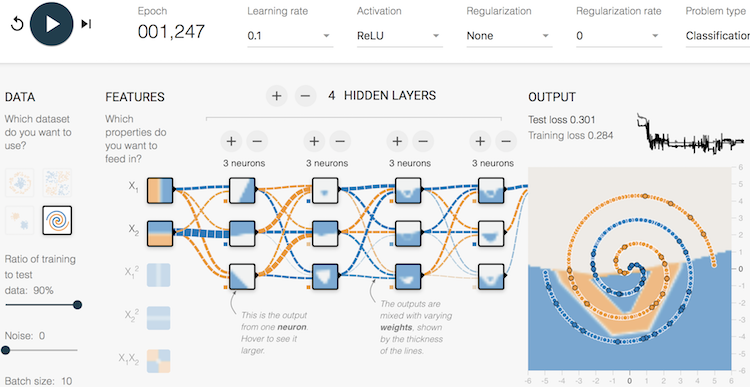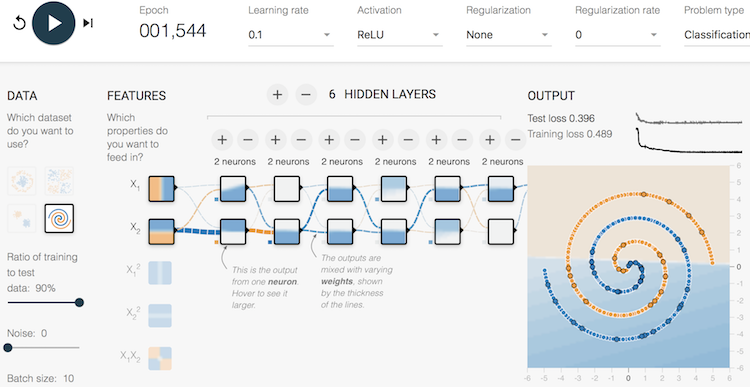Impact of Neural Network Configuration

This section provides a tutorial example to demonstrate the impact of the neuron network configuration on the number of hidden layers and the number of neurons in each hidden layer. Less layers with more neurons per layer seems to be better than more layers with less neurons per layers.

When using a multi-layer neural network, we need to consider its configuration on the number of hidden layers and the number of neurons in each hidden layer. The most frequently asked question is: "Should I use more hidden layers or more neurons in each layer if the total number of neurons is fixed?".

Let's see if we can find the answer by solving the complex classification problem in Deep Playground.

1. Continue with the previous tutorial.

2. Set "Ratio of training to test data" to 90%.

3. Change the hidden layer configuration to 2 layers with 6 neurons in each layer. The total number of neurons is 12.

4. Play the model. It should reach a good solution with no problem.Deep Playground - Complex Model with 2 x 6 Hidden Layers

5. Change the hidden layer configuration to 3 layers with 4 neurons in each layer. The total number of neurons is 12, unchanged. Play the model again. It should reach a poor solution with a test loss of 0.298.Deep Playground - Complex Model with 3 x 4 Hidden Layers

6. Change the hidden layer configuration to 4 layers with 3 neurons in each layer. The total number of neurons is 12, unchanged. Play the model again. It should reach a poor solution with a test loss of 0.301.Deep Playground - Complex Model with 4 x 3 Hidden Layers

7. Change the hidden layer configuration to 6 layers with 2 neurons in each layer. The total number of neurons is 12, unchanged. Play the model again. It fails to reach any solution.Deep Playground - Complex Model with 6 x 2 Hidden Layers

Conclusion, less layers with more neurons per layer seems to be better than more layers with less neurons per layers for a given total number of neurons.